# Add statistical details to charts with ggstatsplot

The `ggstatsplot` package in R is an extension of the ggplot2 package, designed to facilitate the creation of visualizations accompanied by statistical details.
This post showcases the key features of `ggstatsplot` and provides a set of graph examples using the package.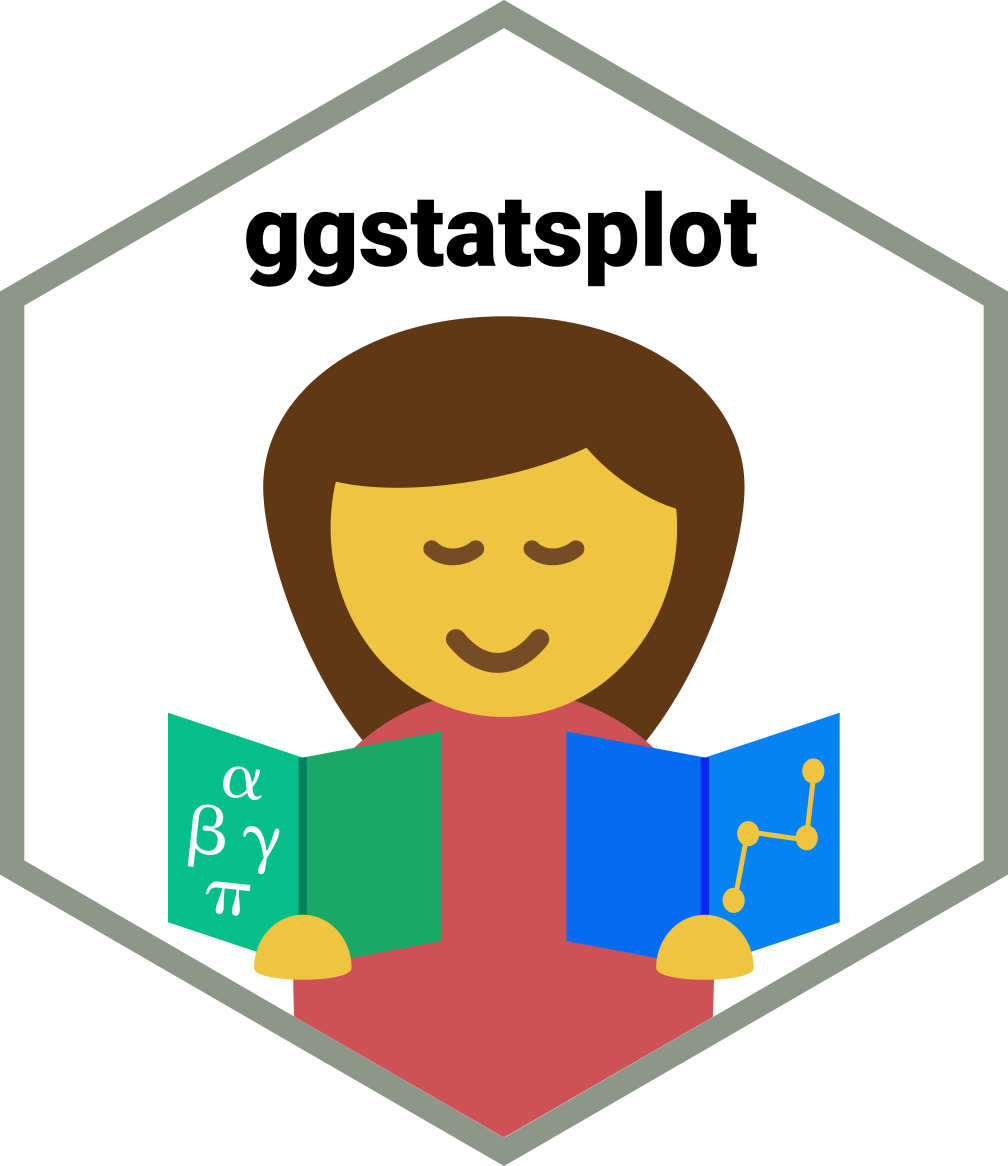{ggstatsplot}

# Quick start

The `ggstatsplot` package in R is an extension of the ggplot2 package, designed to facilitate the creation of visualizations accompanied by relevant statistical details.

It streamlines the process of integrating statistical tests with informative plots, making it easier for researchers and data analysts to communicate their findings effectively.

✍️ author → Indrajeet Patil

📘 documentationgithub

⭐️ more than 1000 stars on github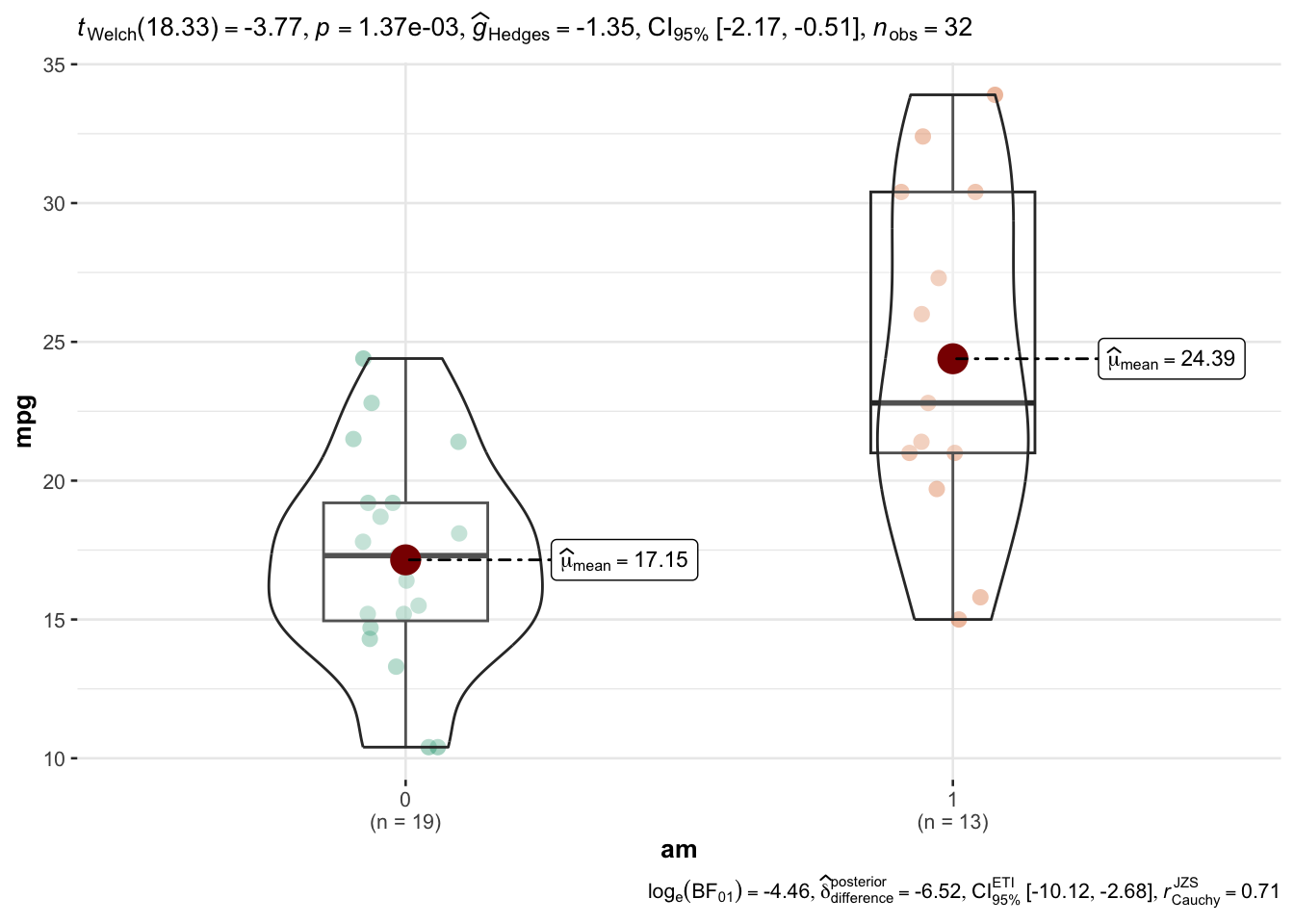``````library(ggstatsplot)
ggstatsplot::ggbetweenstats(data = mtcars, x = am, y = mpg, type = "p")``````

# Installation

Getting started with `ggstatsplot` is straightforward.

First, ensure you have `ggplot2` installed. Then, you can install `ggstatsplot` directly from CRAN using the `install.packages` function:

``install.packages("waffle", repos = "https://cinc.rud.is")``

# Basic usage

The `ggstatsplot` package comes with about 9 functions, each of them targeting a specific statistical test.

For instance, the `ggscatterstats()` function visualizes the relationship between 2 variables `x` and `y` using a scatterplot. It runs a linear regression and draw a regression line that provides a visual representation of the linear relationship between the two variables. The shaded region around it represents the confidence interval.

The marginal histograms on the top and right side of the plot show the distribution of the `x` and `y` variables, respectively. Additionally, the plot provides statistical details like correlation coefficient, p-value, and sample size.

Here is an example using the famous `mtcars` dataset, checking the relationship between the `hp` and `mpg` columns:

``ggscatterstats(data = mtcars, x = hp, y = mpg)``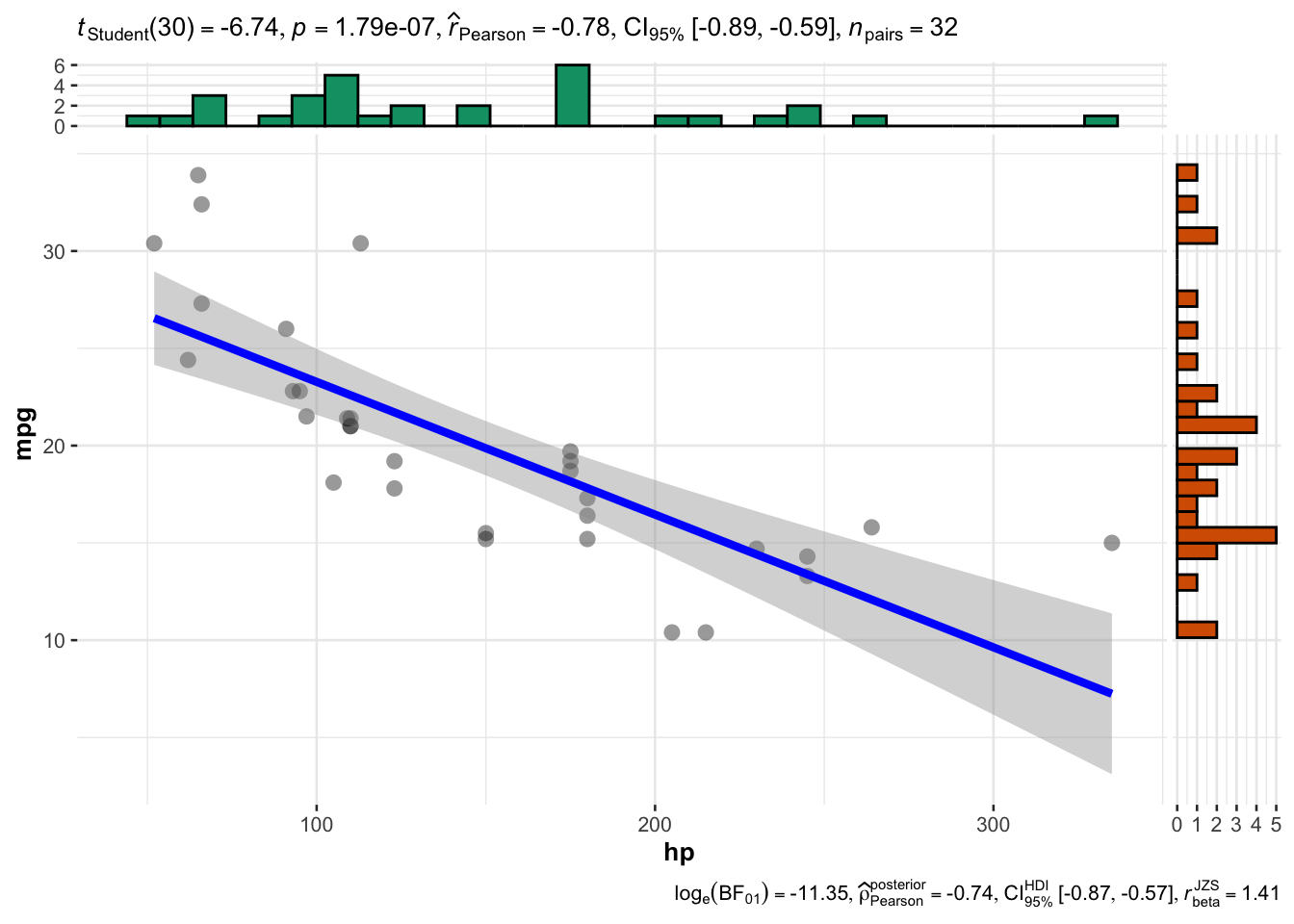Now, let’s try to summarize the power of `ggstatsplot` through its main functions:

# Key features

Here is an overview of the main function offered by ggstatsplot with a short description of what they do:

## → ggbetweenstats

`ggbetweenstats()` creates violin plots for comparisons between groups or conditions, accompanied by results from statistical tests.

Example:

``ggstatsplot::ggbetweenstats(data = mtcars, x = am, y = mpg, type = "p")``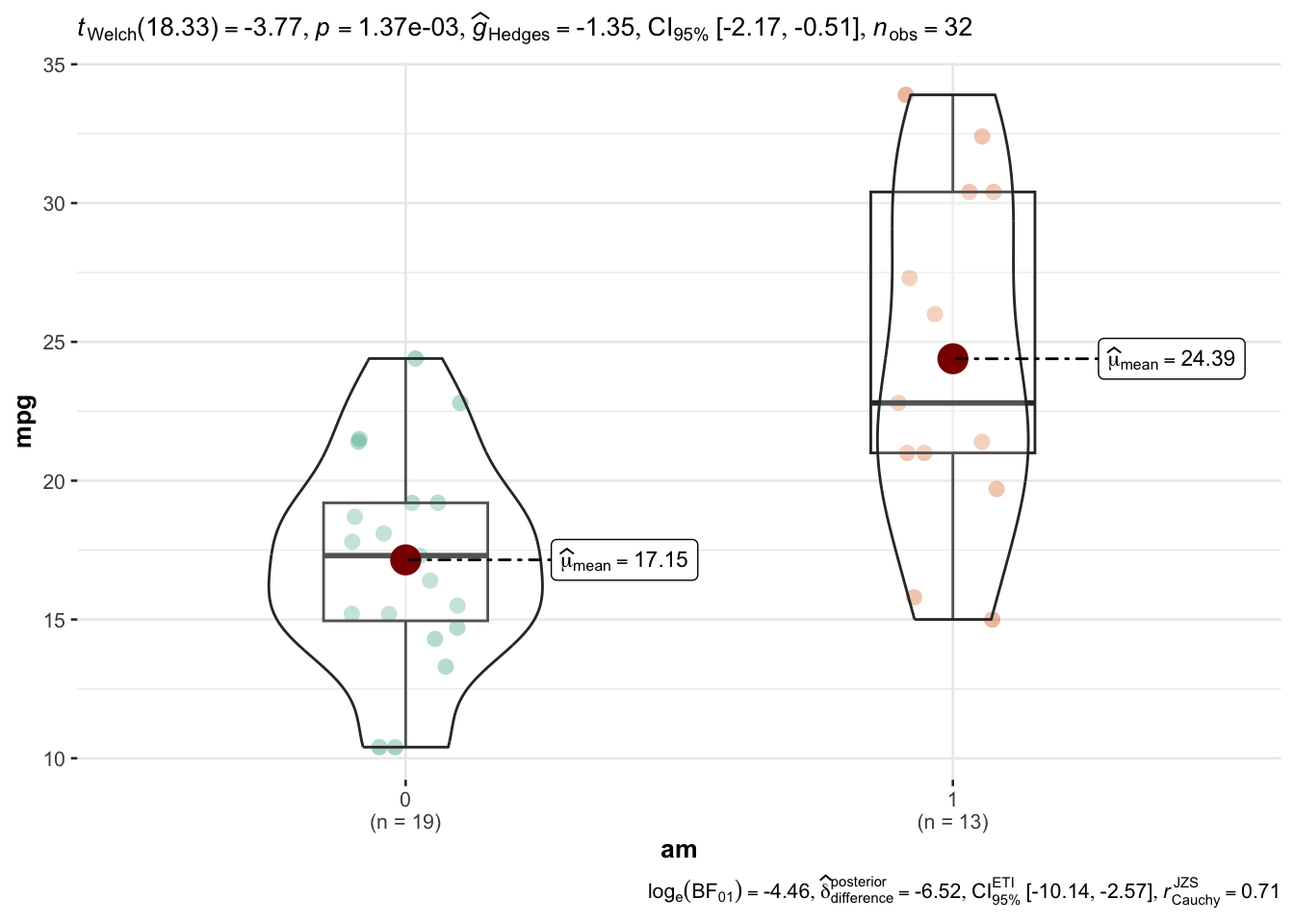## → ggwithinstats

`ggwithinstats()` is used to display data distributions, descriptive statistics, and statistical tests for different groups within the same variable.

The function is particularly useful for visualizing and testing differences within a single categorical variable.

Here’s a simple example using the mtcars dataset that comes built-in with R:

``````ggwithinstats(
data = bugs_long,
x = condition,
y = desire,
type = "nonparametric", ## type of statistical test
xlab = "Condition", ## label for the x-axis
ylab = "Desire to kill an artrhopod", ## label for the y-axis
package = "yarrr", ## package from which color palette is to be taken
palette = "info2", ## choosing a different color palette
title = "Comparison of desire to kill bugs",
caption = "Source: Ryan et al., 2013"
) + ## modifying the plot further
ggplot2::scale_y_continuous(
limits = c(0, 10),
breaks = seq(from = 0, to = 10, by = 1)
)``````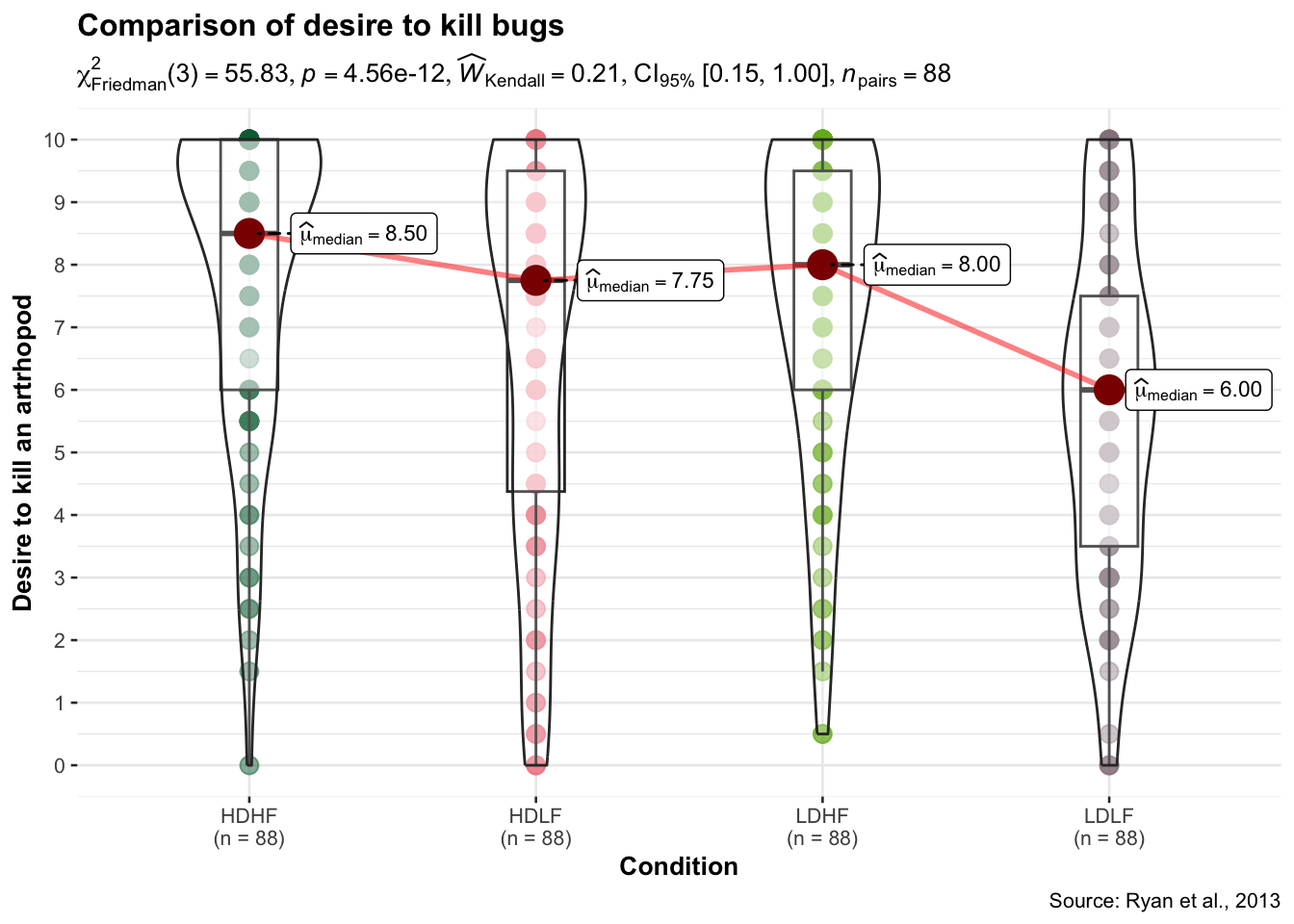## → gghistostats

`gghistostats()` generates histograms to visualize the distribution of a numeric variable and checks if its mean is significantly different from a specified value with a one-sample test:

``````gghistostats(
data       = ggplot2::msleep,
x          = awake,
title      = "Amount of time spent awake",
test.value = 12,
binwidth   = 1
)``````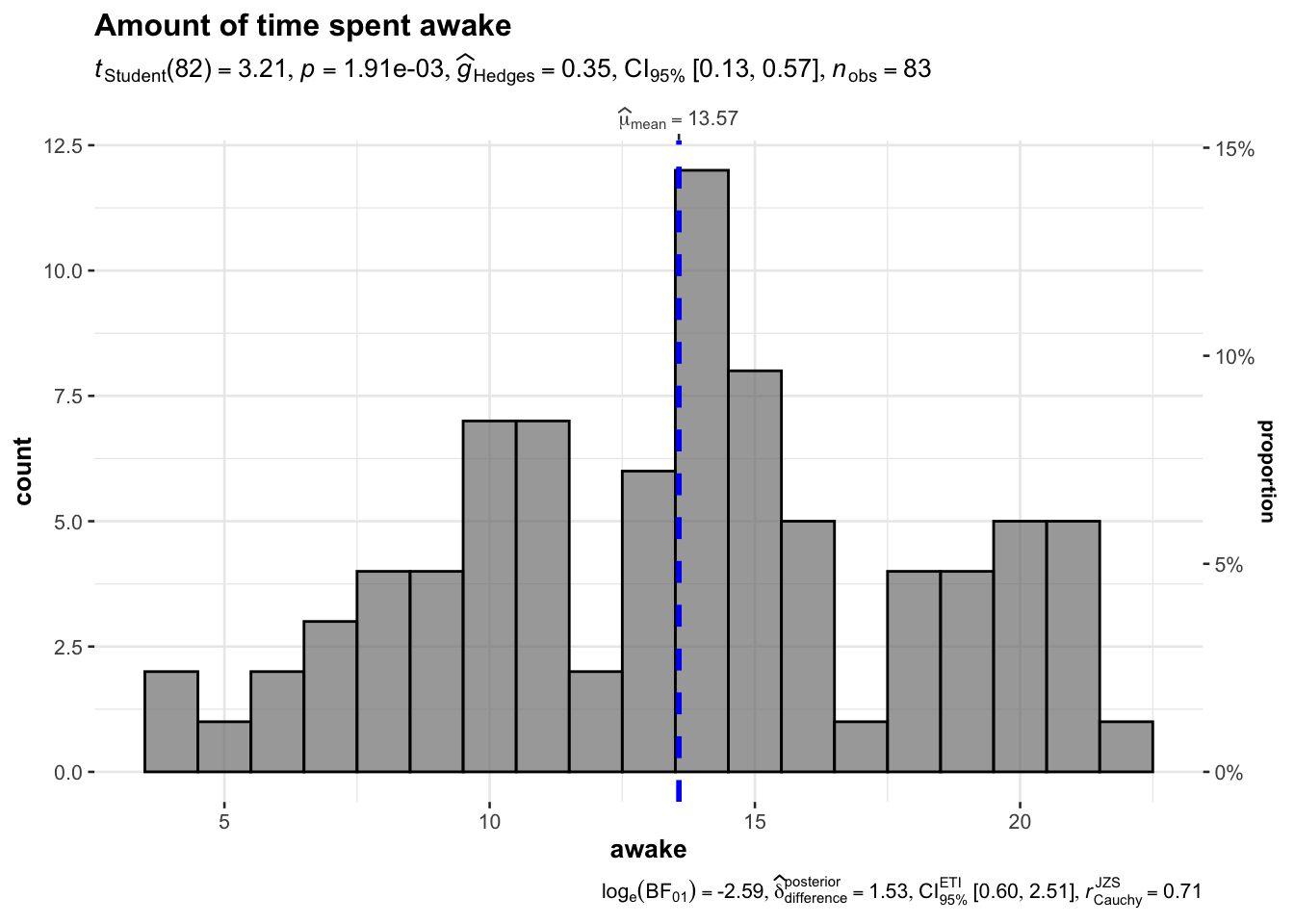## → Other functions

Several other functions are available: - `ggdotplotstats()` → Similar to `gghistostats()`, but intended for labeled numeric variables.

• `ggscatterstats()` → Creates a scatterplot with marginal distributions overlaid on the axes and results from statistical tests in the subtitle.

• `ggcorrmat()` → Produces a correlalogram (a matrix of correlation coefficients) with statistical details.

• `ggpiestats()` → Creates a pie chart for categorical or nominal variables with results from contingency table analysis included in the subtitle.

• `ggbarstats()` → An alternative to `ggpiestats()`, this function creates bar charts for categorical data with associated statistical tests.

• `ggcoefstats()` → Generates dot-and-whisker plots for regression models and meta-analysis.

Those functions are described more in depth in other pages of the R graph gallery.

Related chart types

## Contact

This document is a work by Yan Holtz. Any feedback is highly encouraged. You can fill an issue on Github, drop me a message on Twitter, or send an email pasting yan.holtz.data with gmail.com.